0

# 【数据结构 | 入门】 入坑篇 （浙江大学数据结构学习笔记）🤵‍♂️ 个人主页: @计算机魔术师
👨‍💻 作者简介：CSDN内容合伙人，全栈领域优质创作者。

## 一、什么是数据结构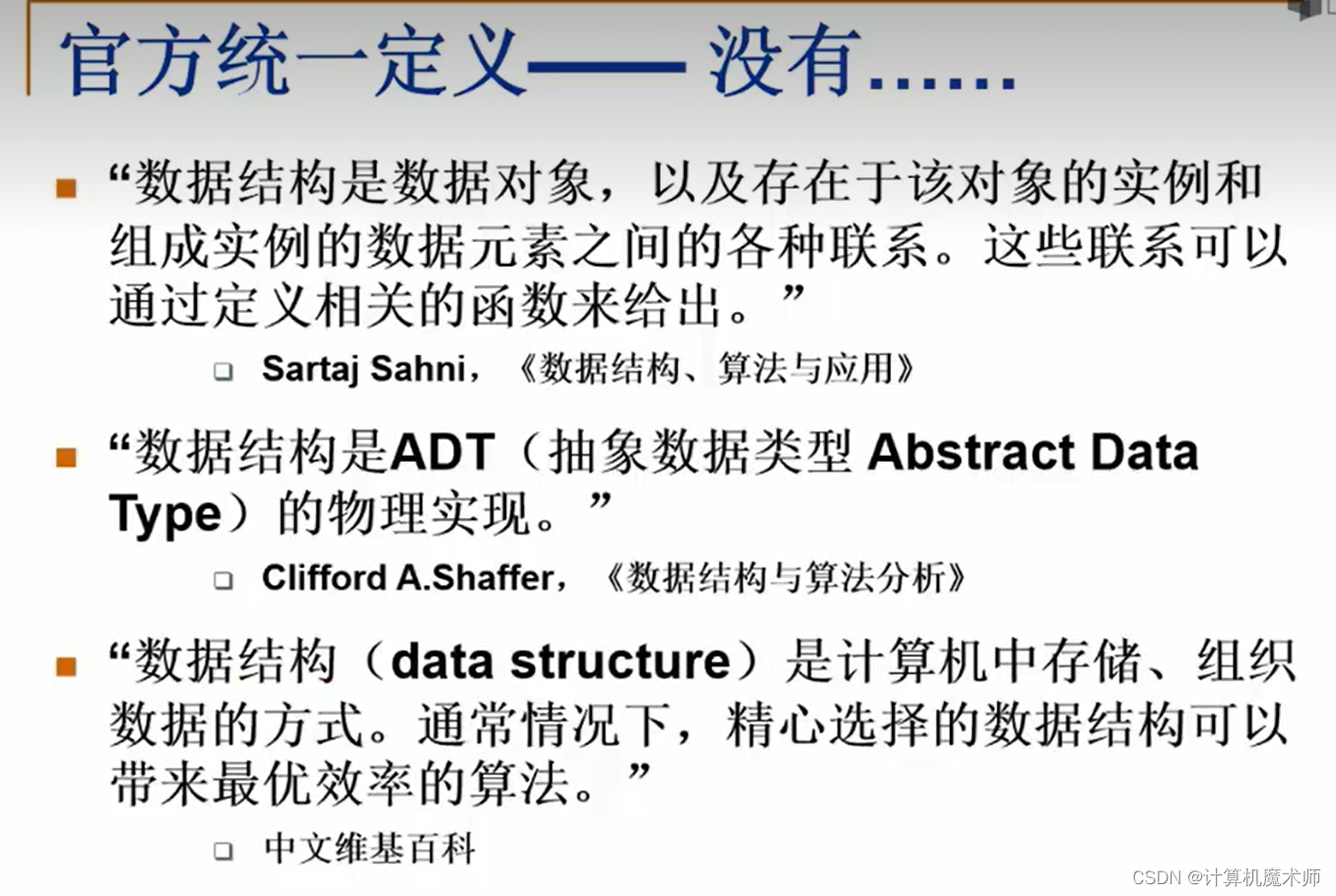1. 方法1： 随便放 但是查找的时候非常麻烦！
2. 方法2： 按照书名的拼音字母顺序排放 查找的时候就可以使用 `二分查找!! ` （ 也叫截半查找） 问题：插入新书，需要移动很大一部分书
3. 实际上，我们在图书馆都是按照分科分别区域，每个分科按照书名的拼音字母顺序 好处：大大降低规模，插入于查找都小了很多

## 二、简单循环例子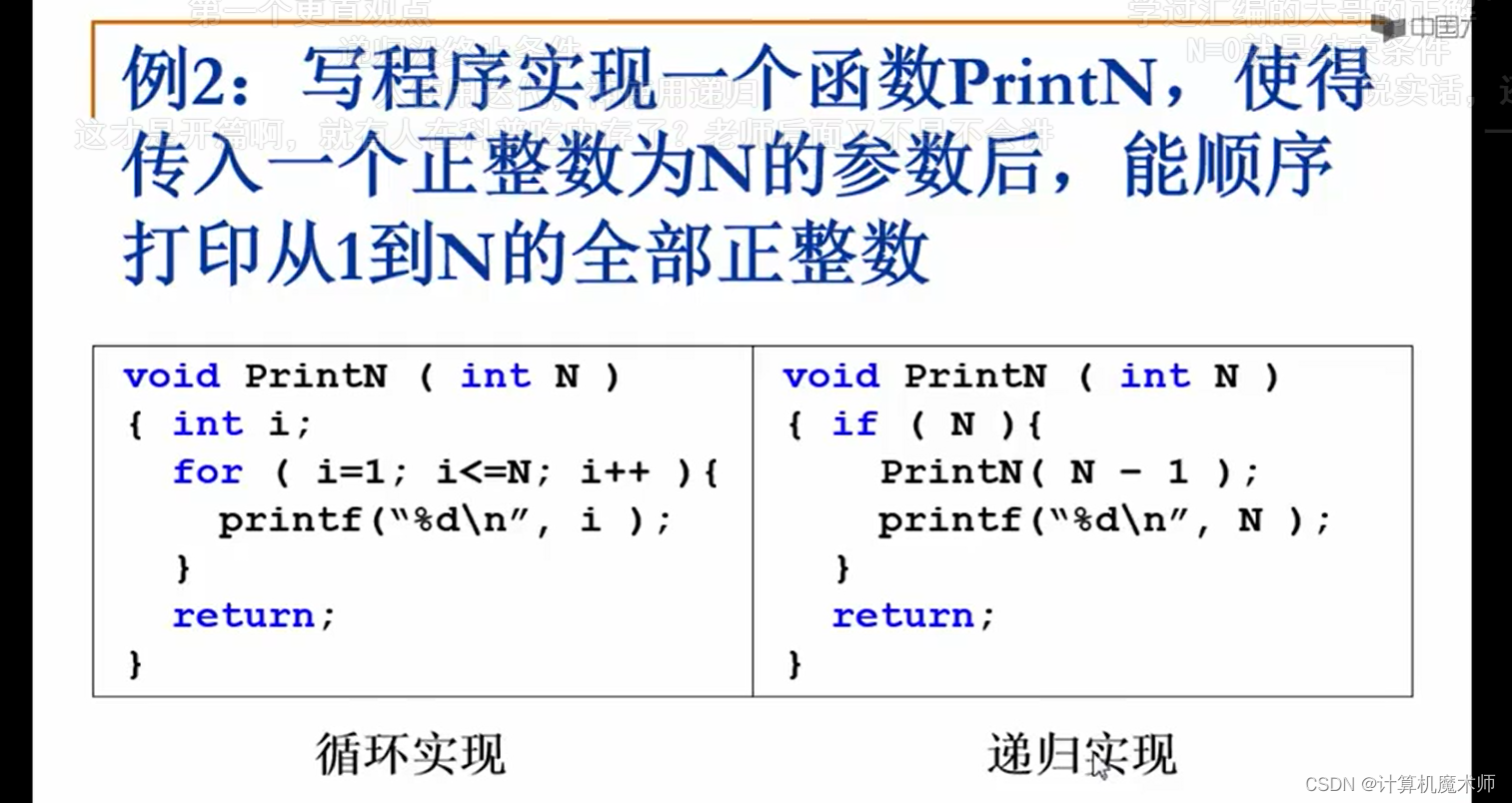1. 循环实现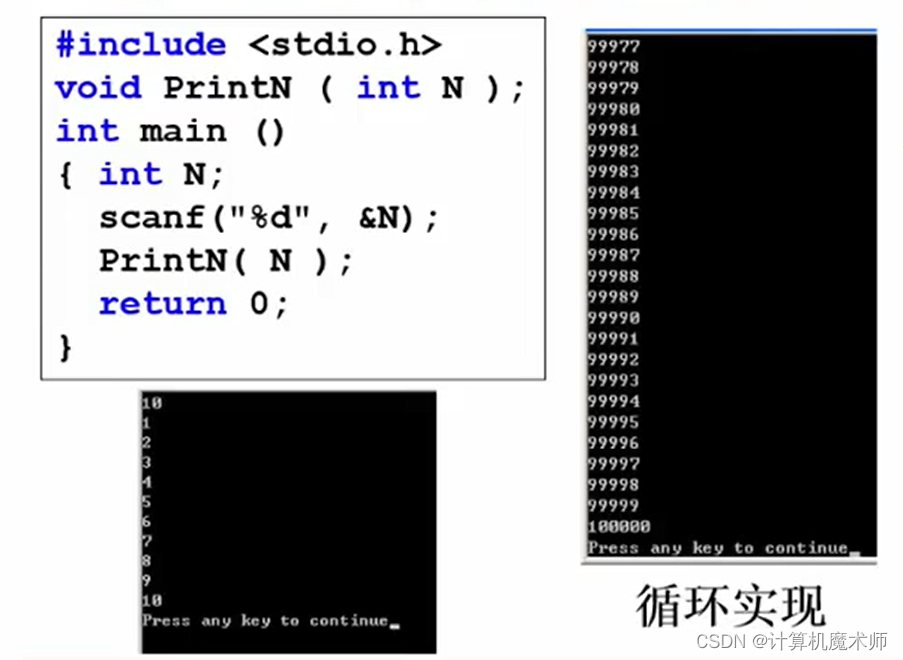可以看到，程序慢慢打印结果，而
2. 递归实现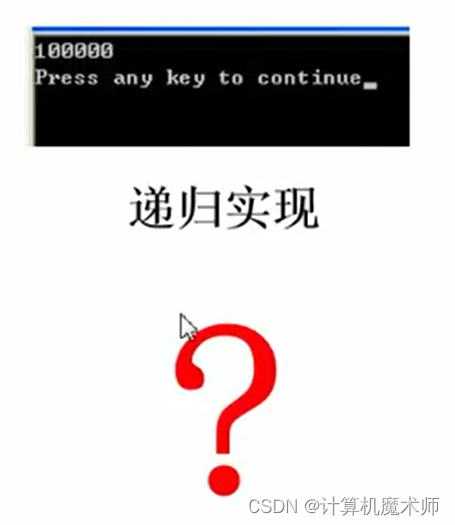## 三、多项式例子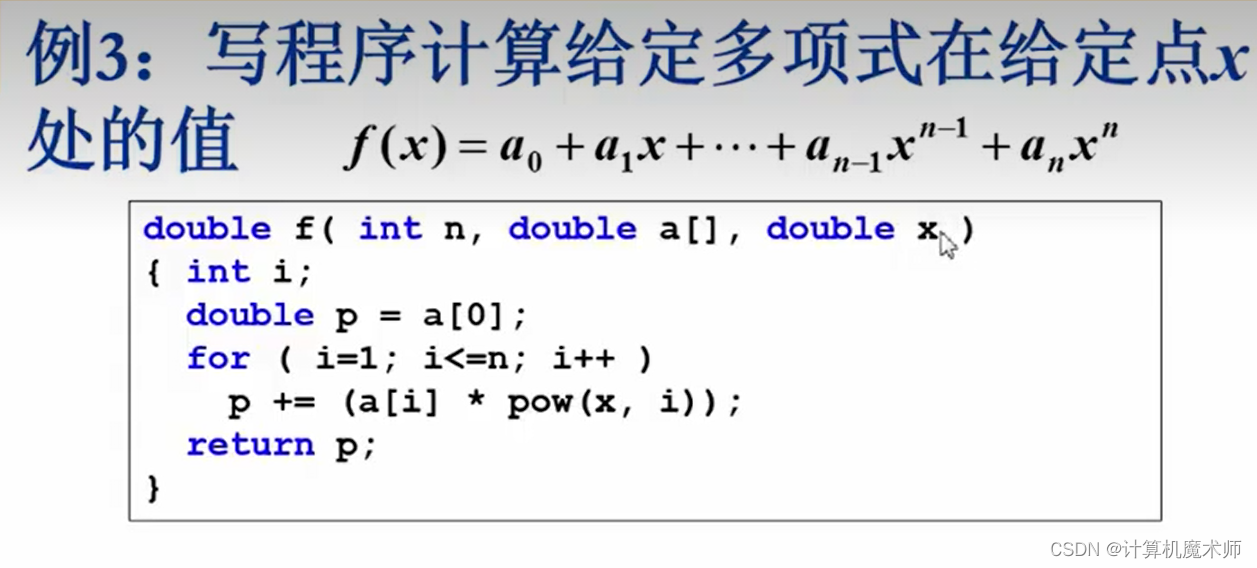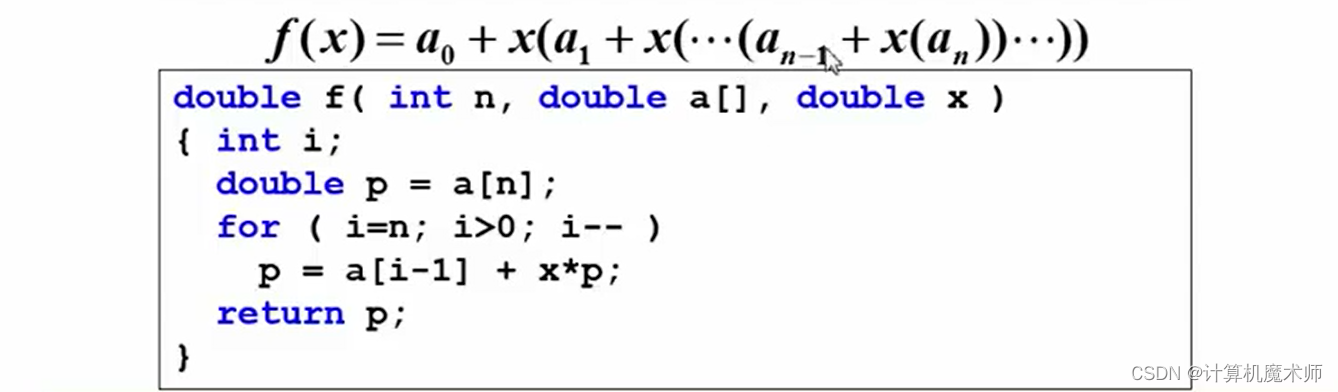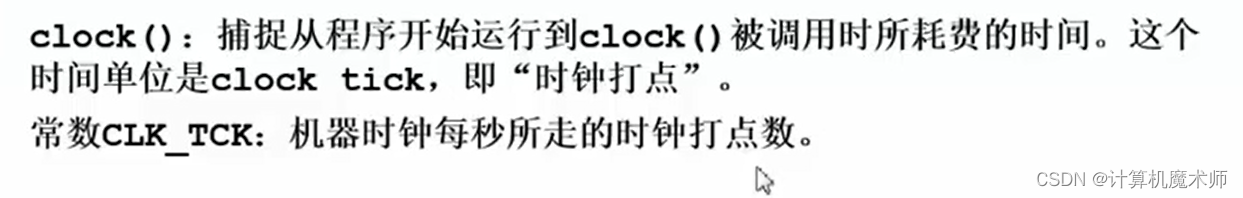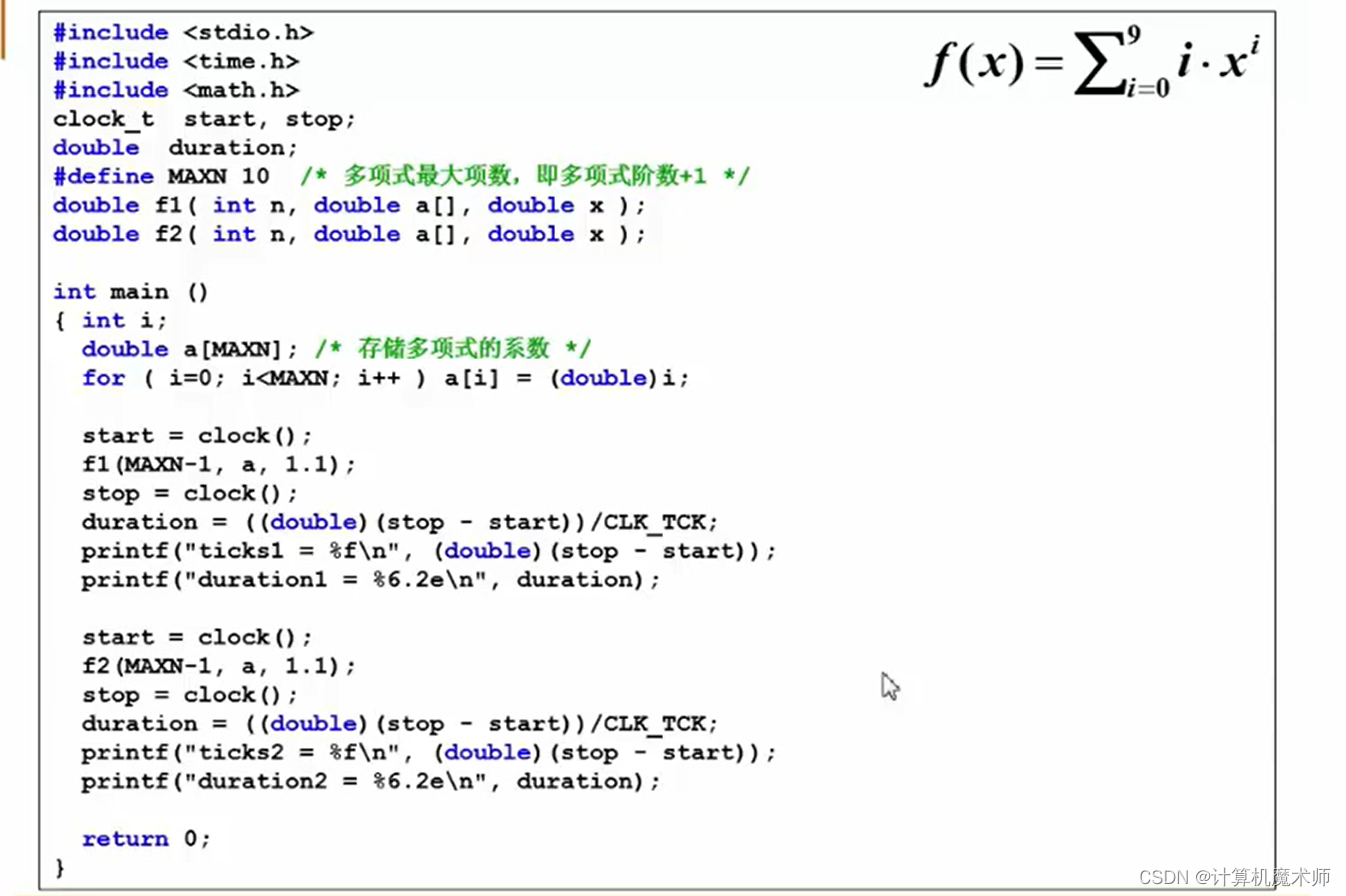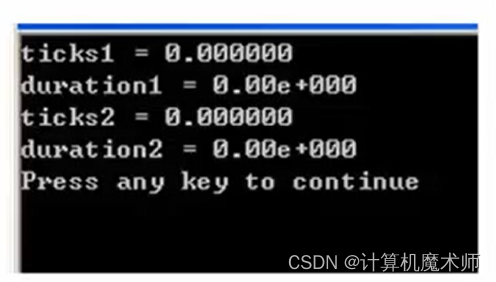``````tick
``````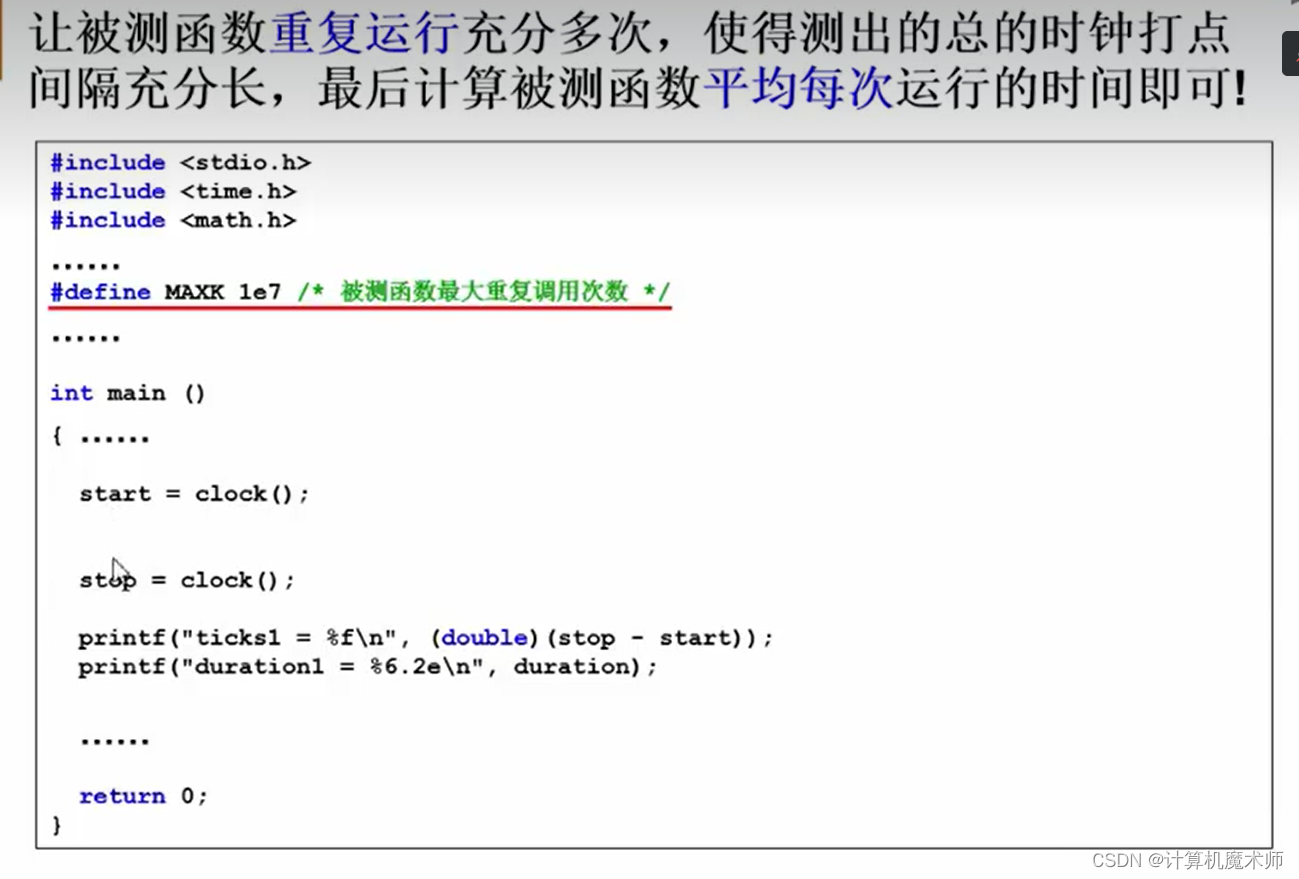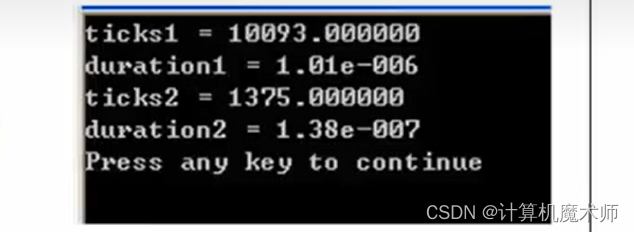## 四、数据结构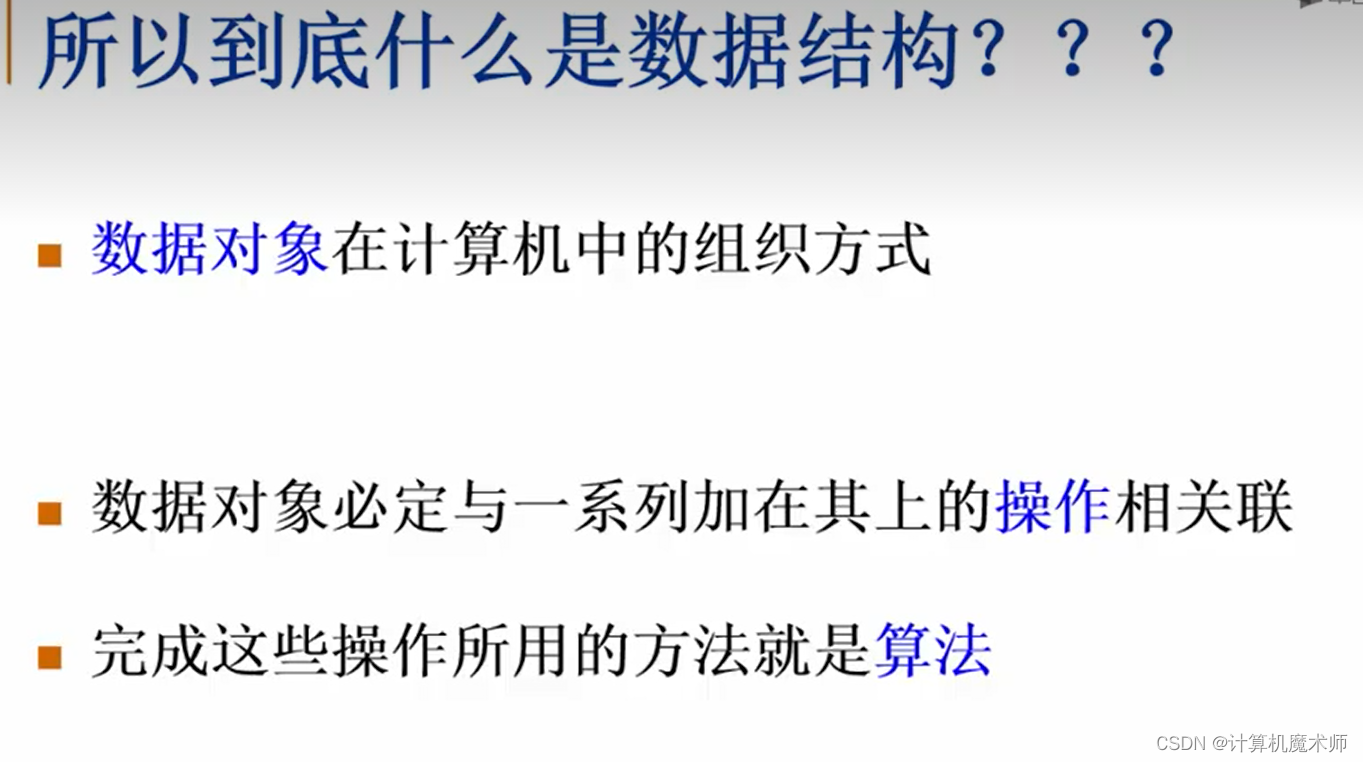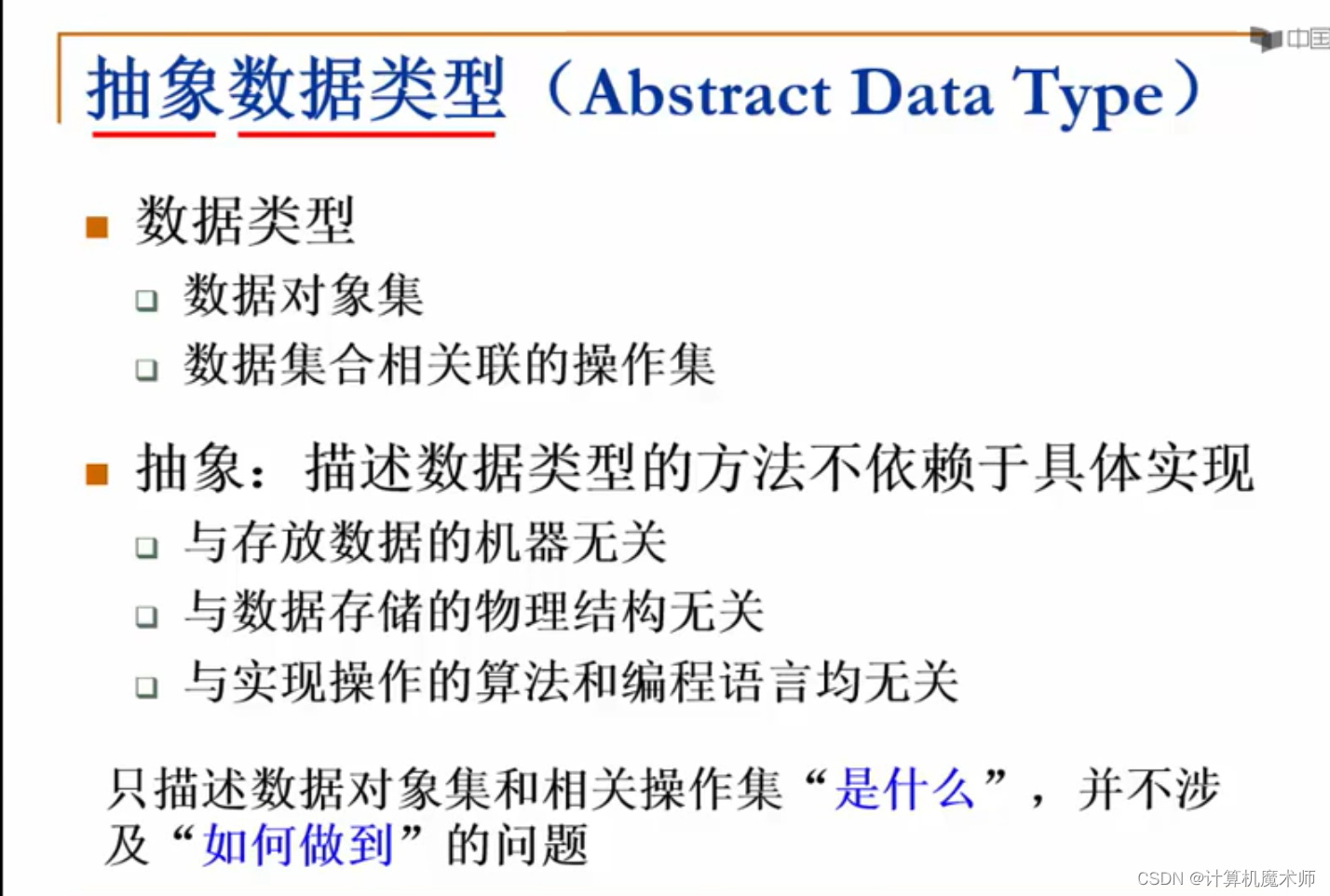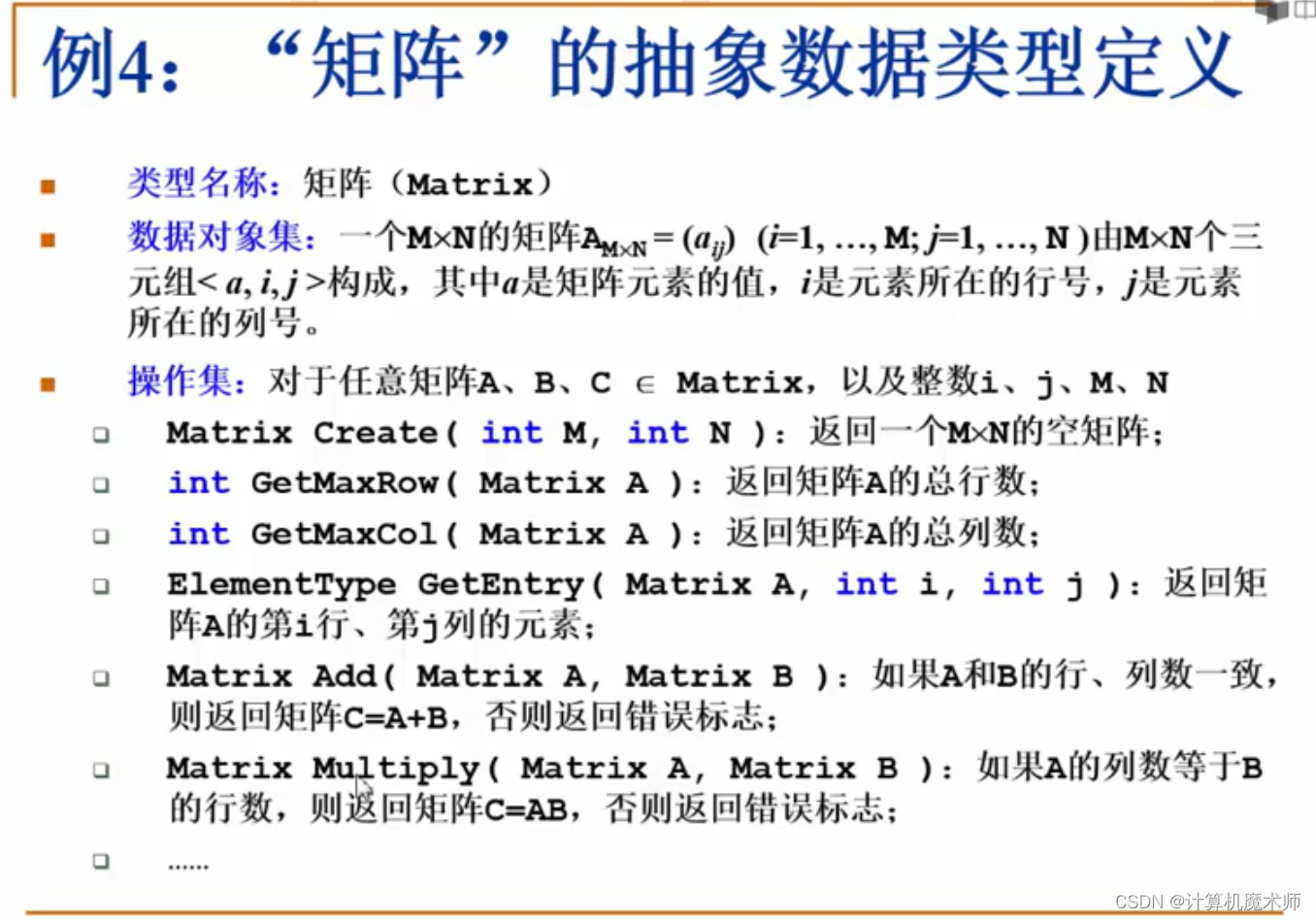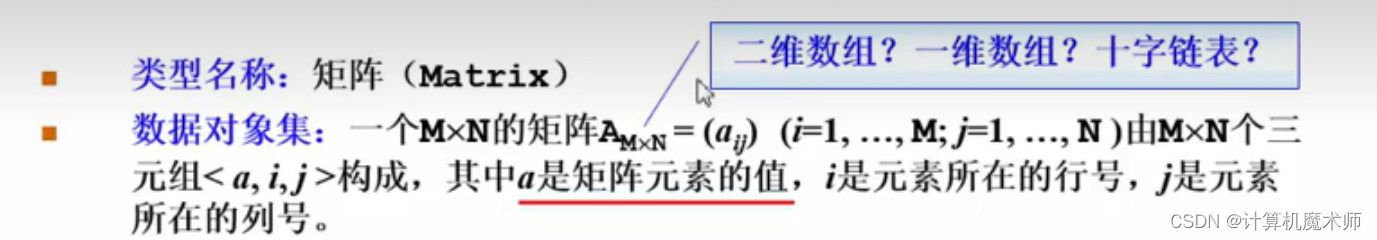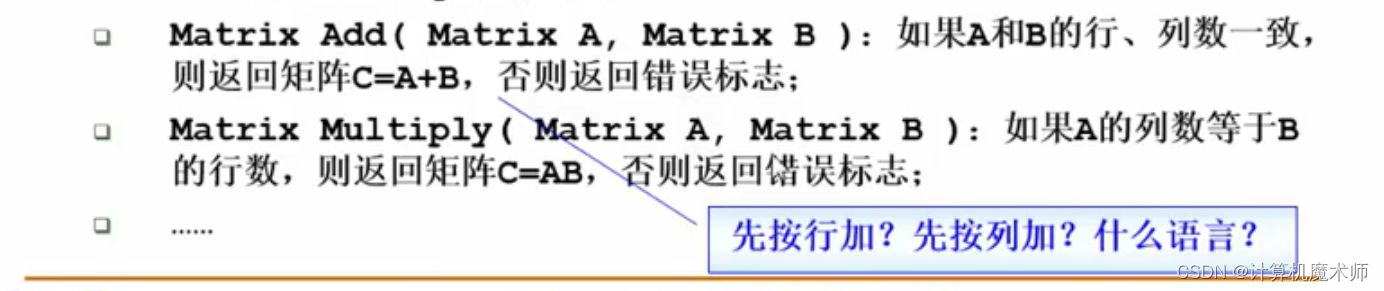## 五、算法

``````algorithm
``````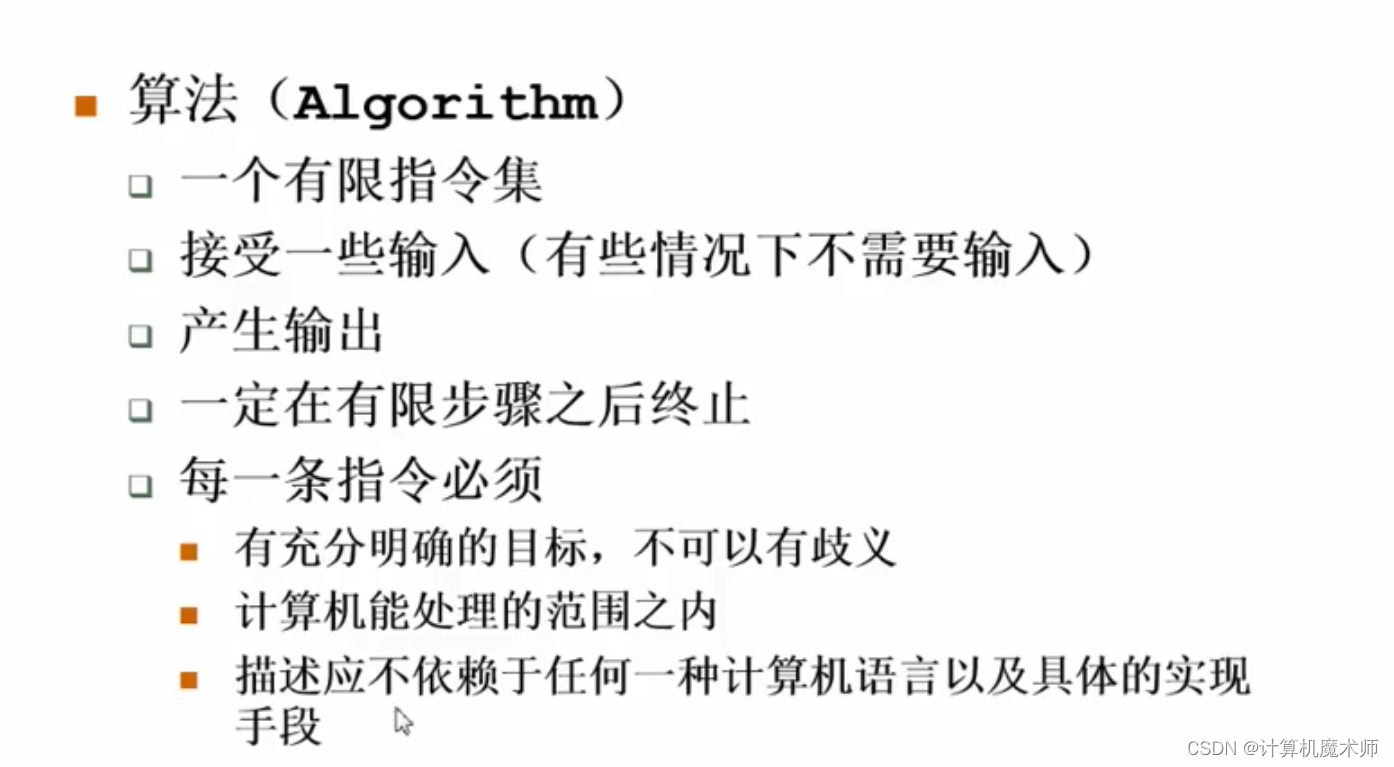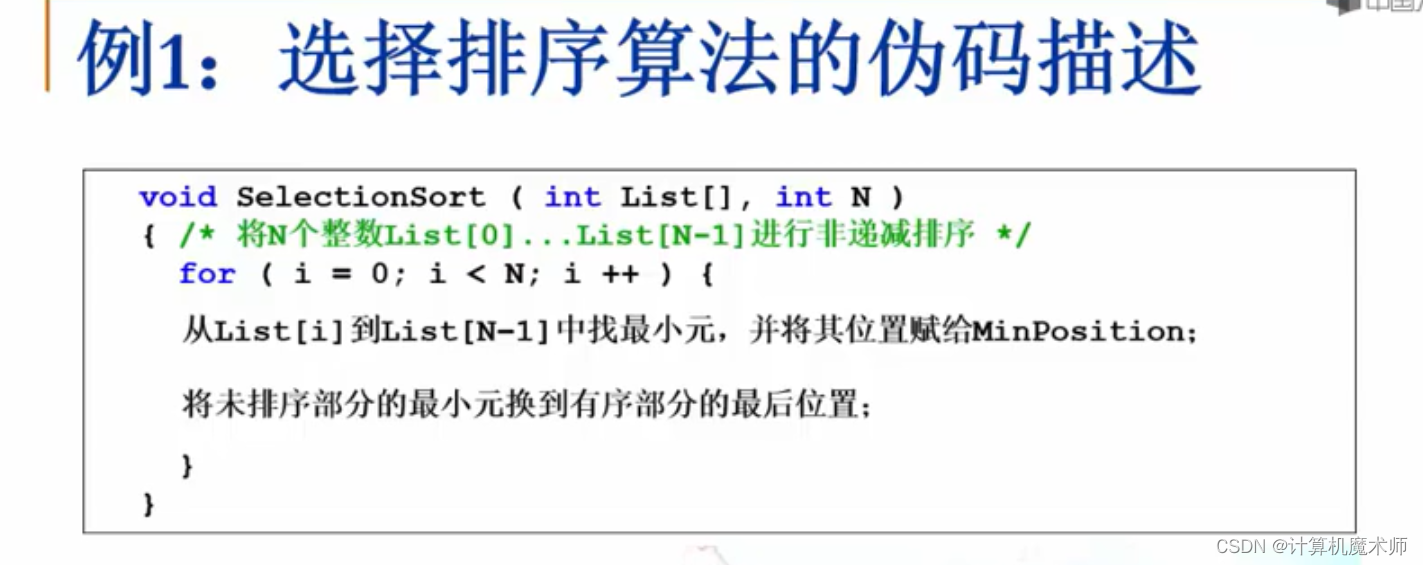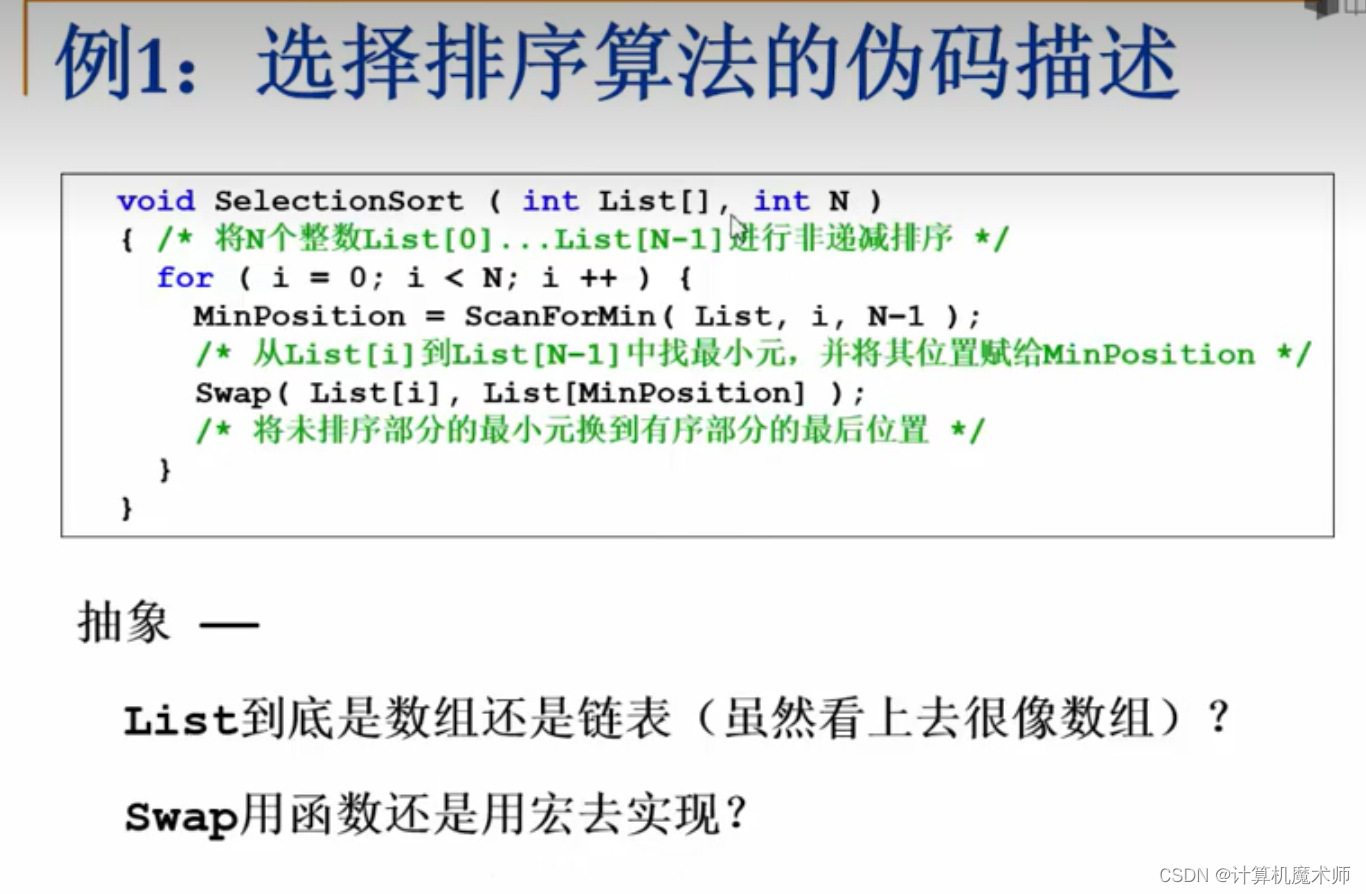## 5.1 描述算法好坏

``````s(n)
``````

``````t(n)
``````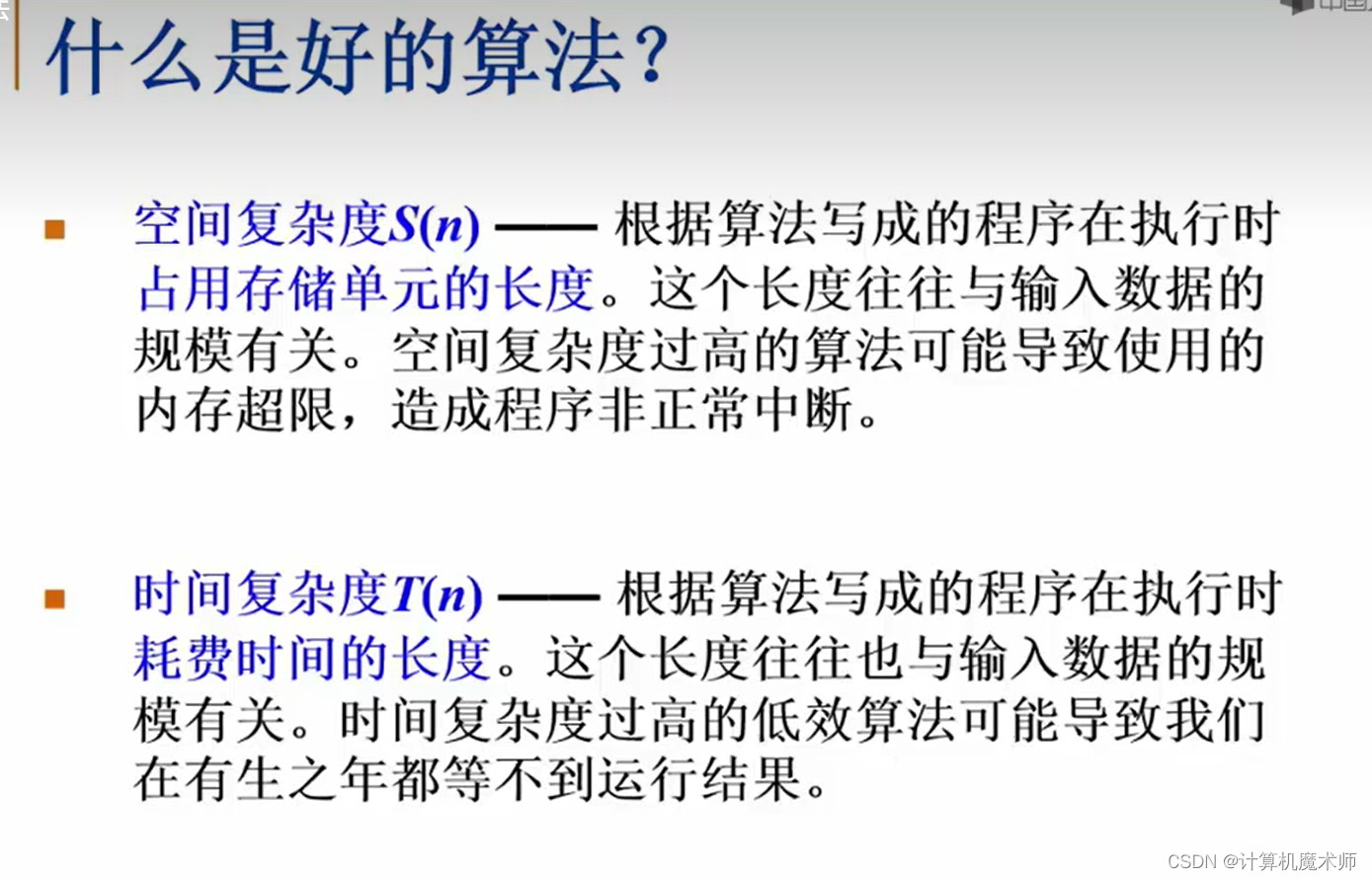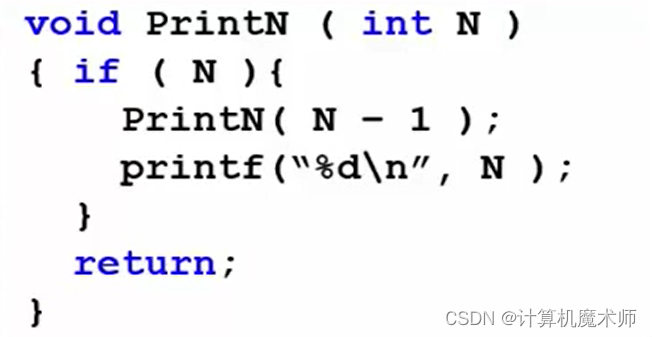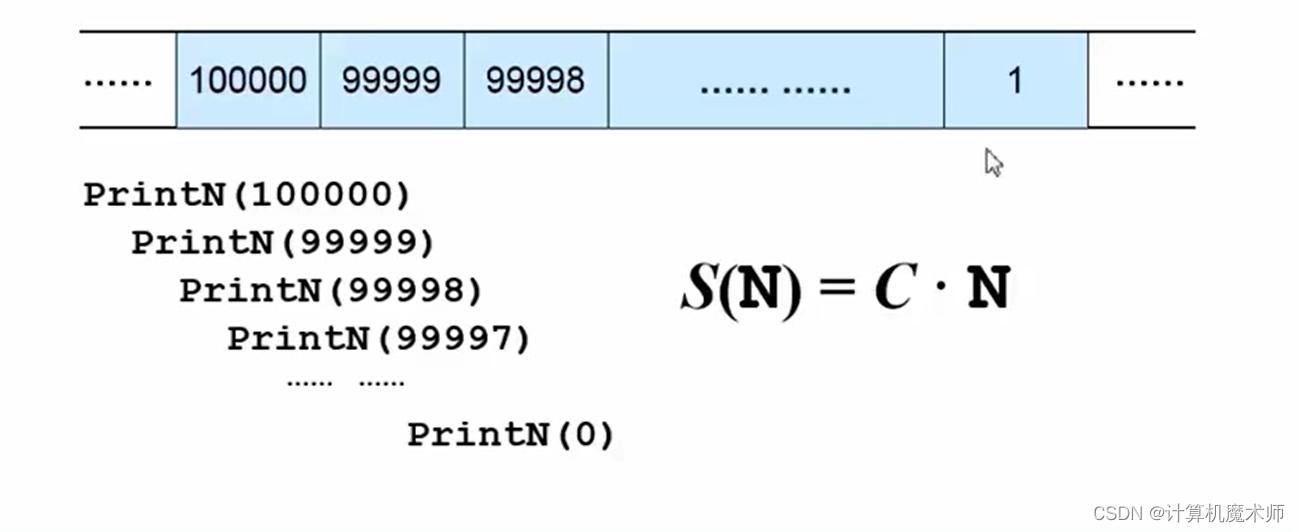``````    s

(

n

)

=

c

∗

n

s(n)=c*n

s(n)=c∗n
``````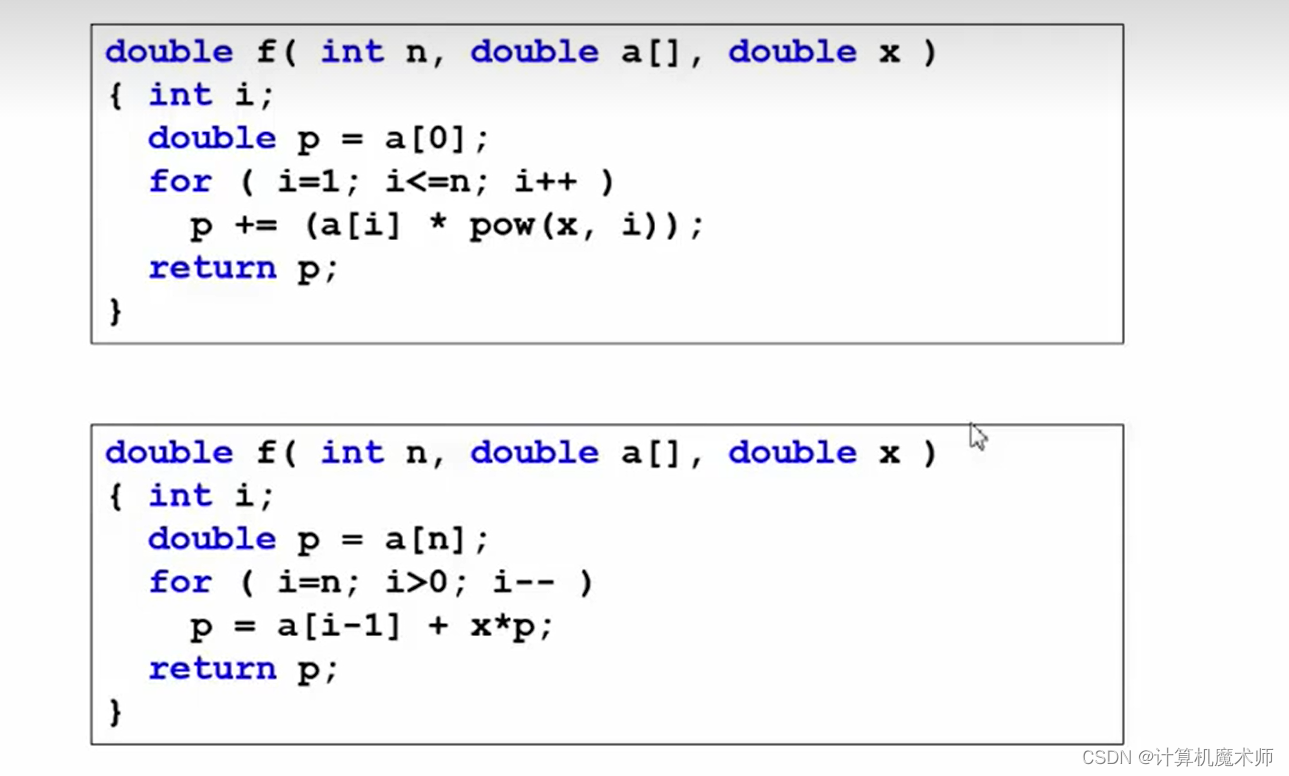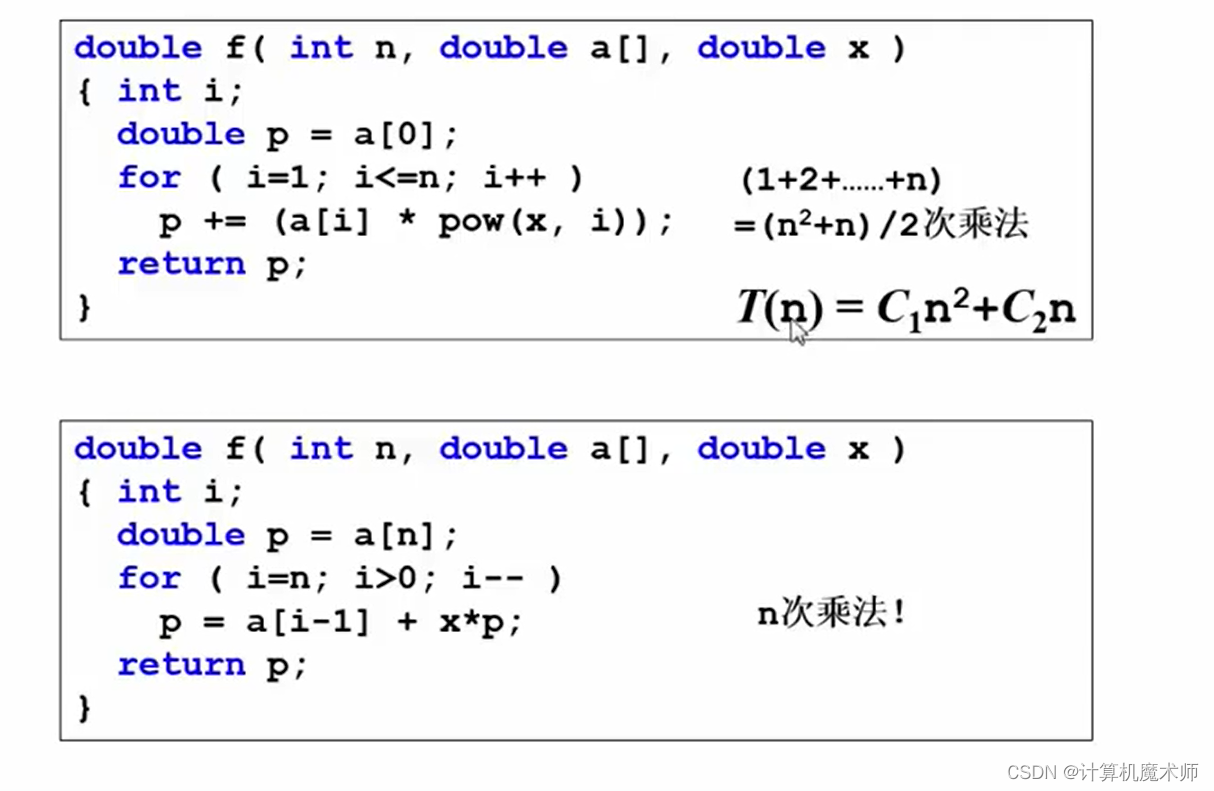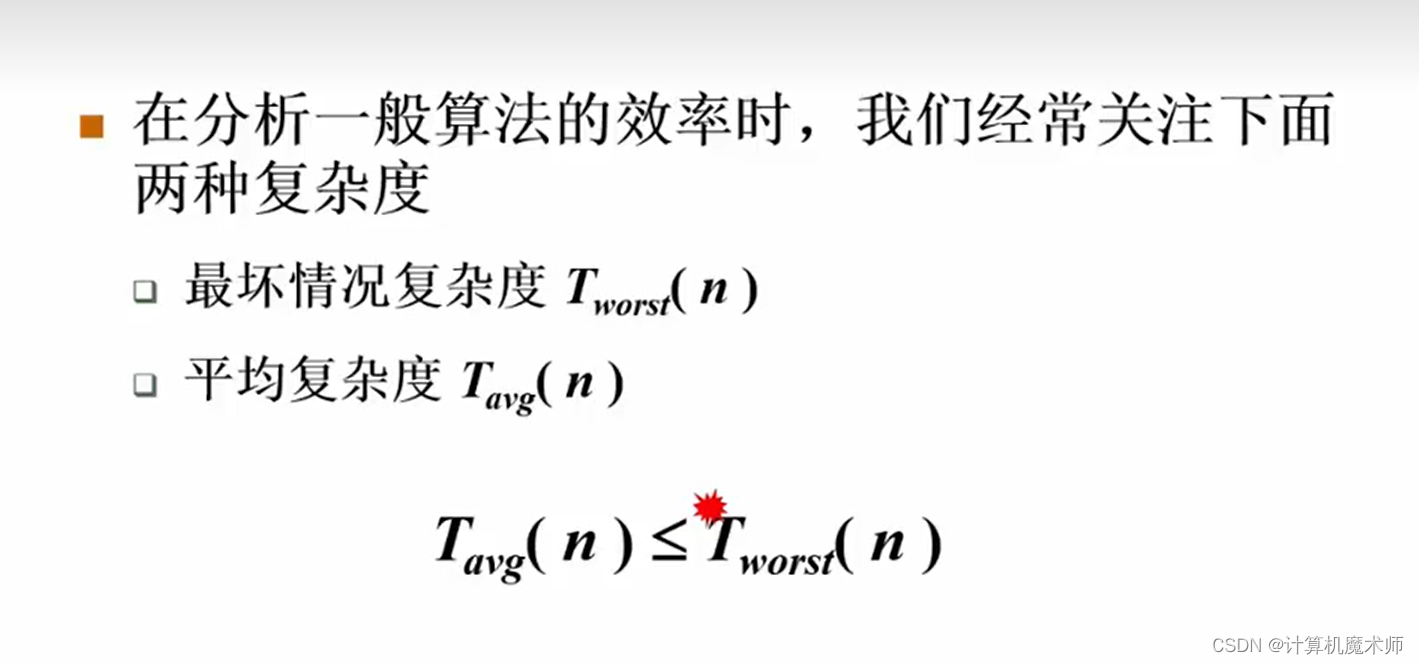## 5.2 复杂度的渐进表示法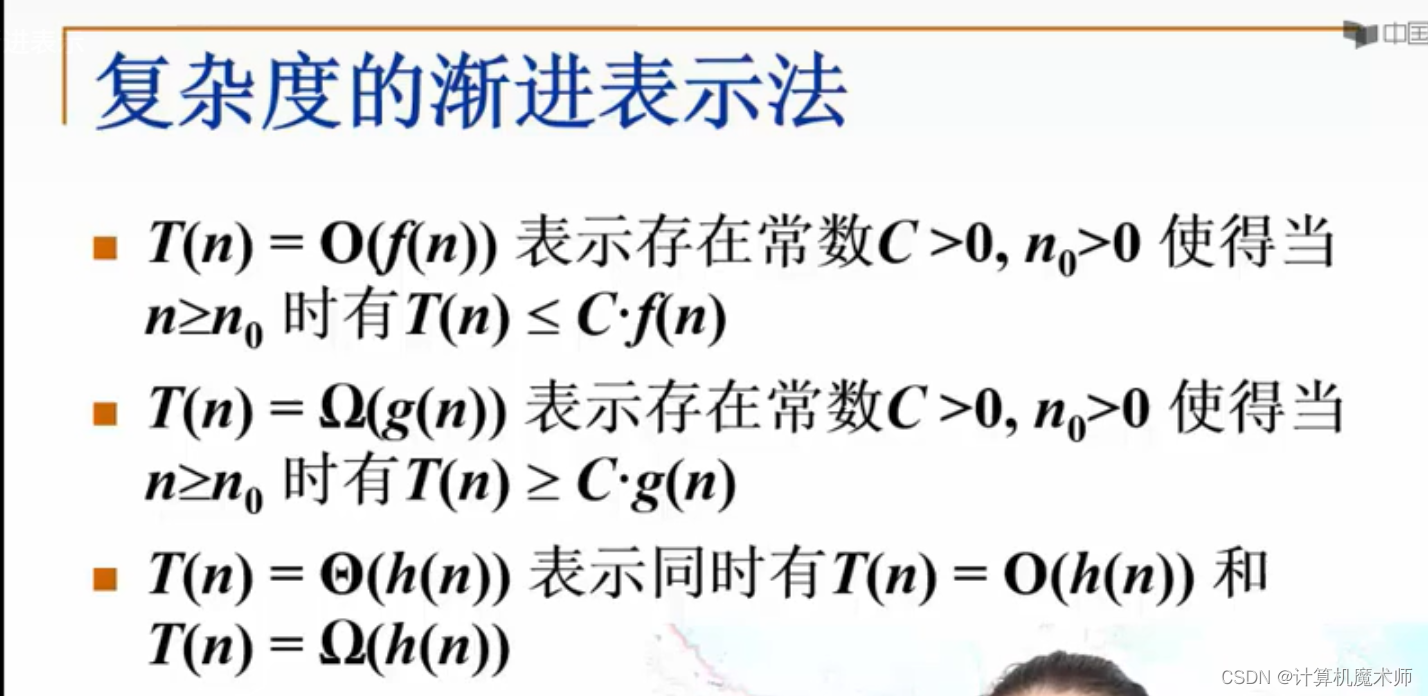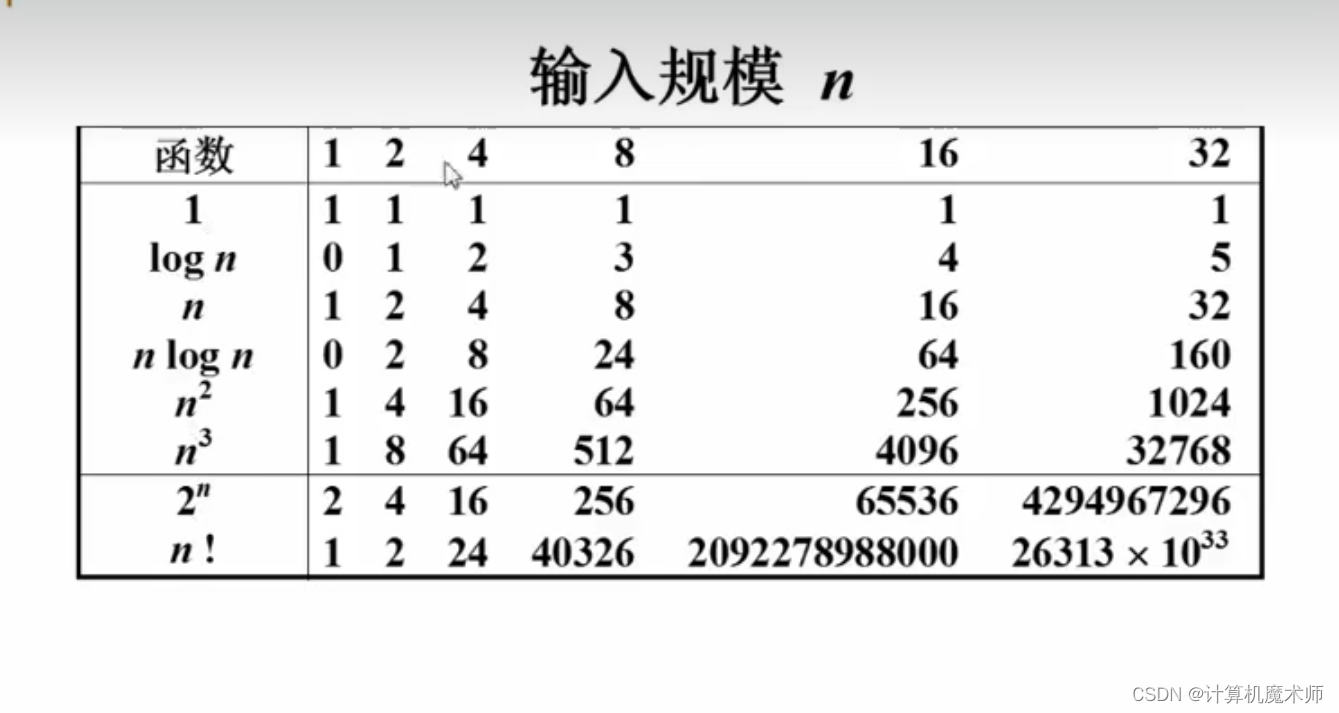``````    l

o

g

n

log n

logn 来说显然是最好的，增长最慢，其下标是2还是10并没有影响，只是一个倍数变化
``````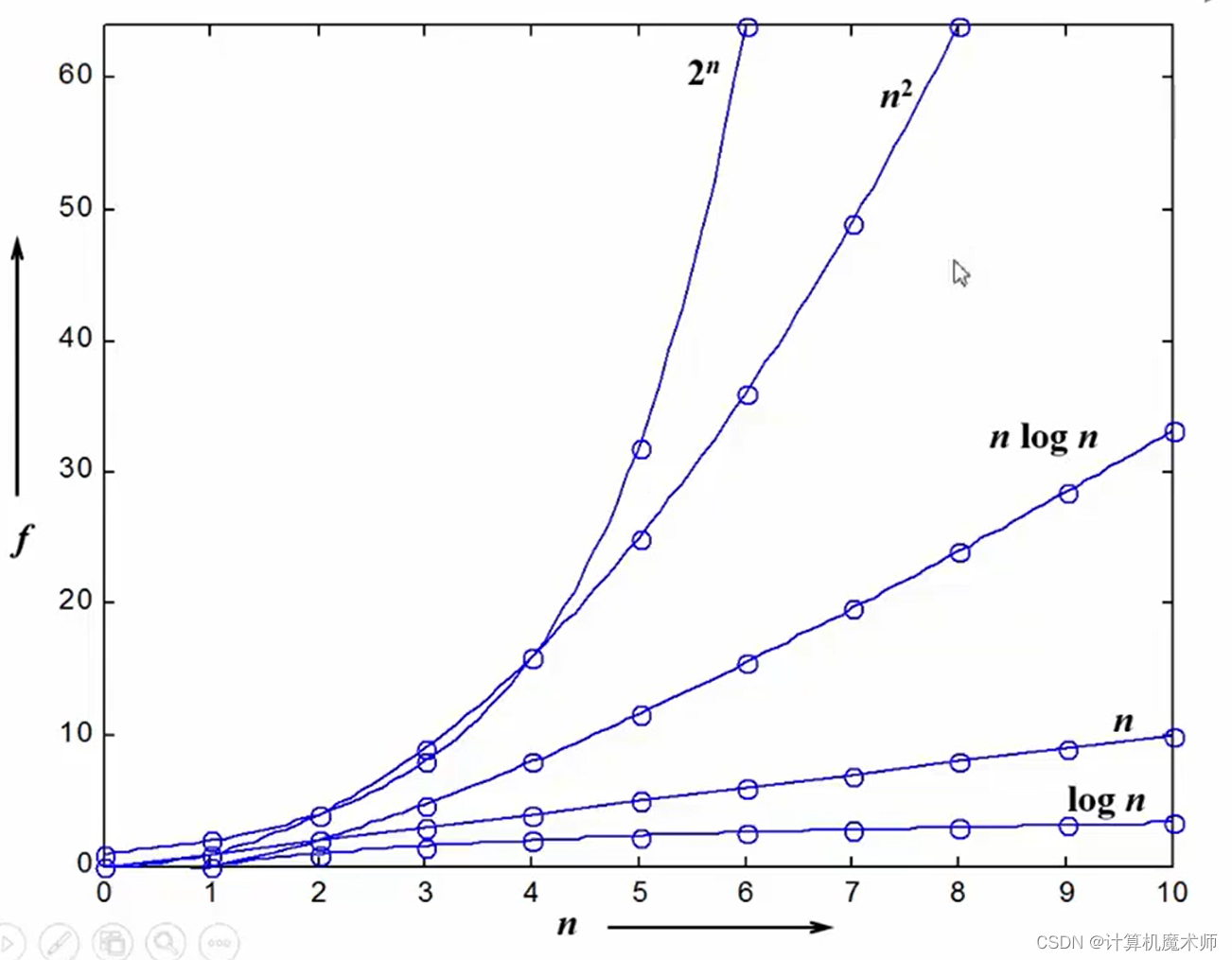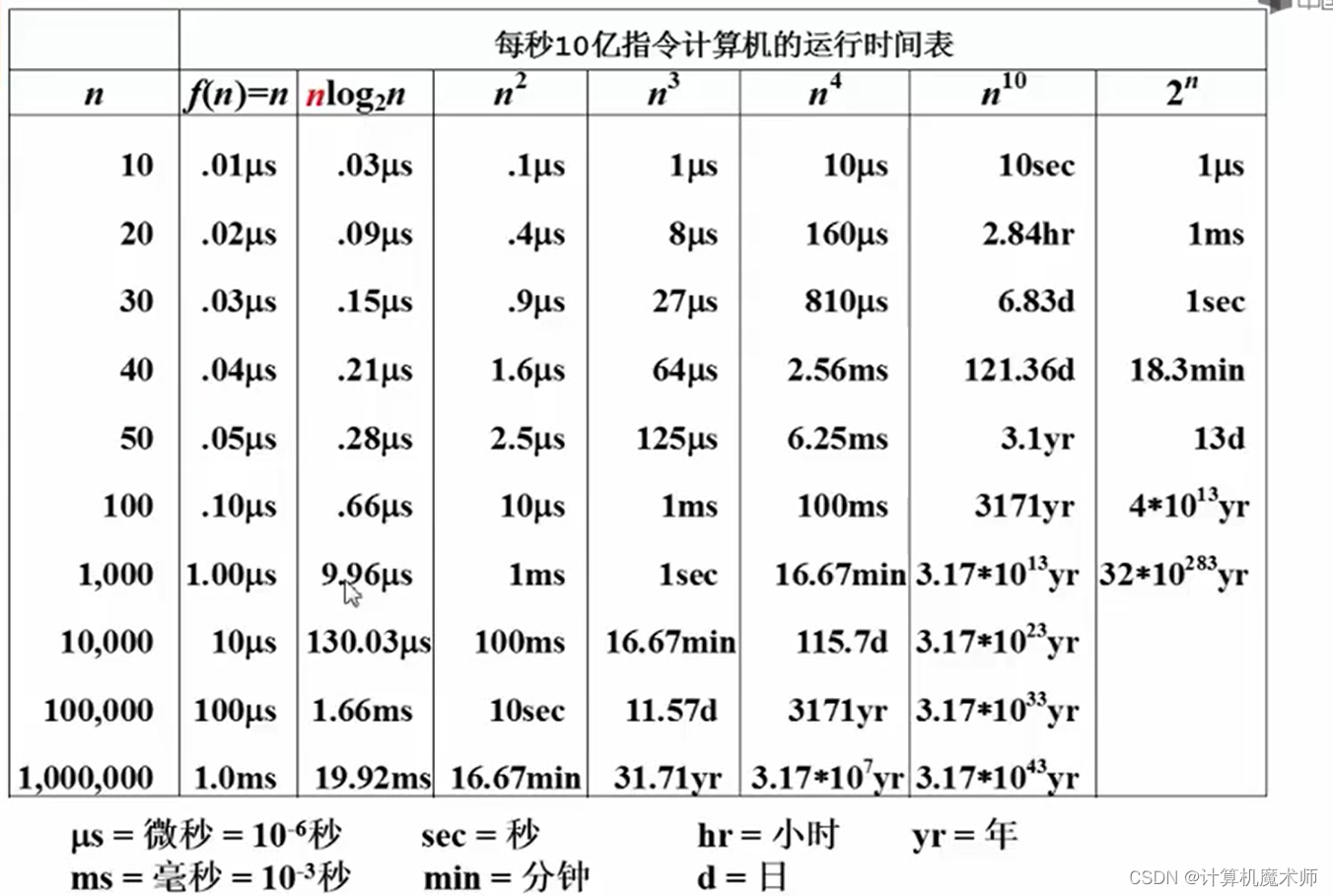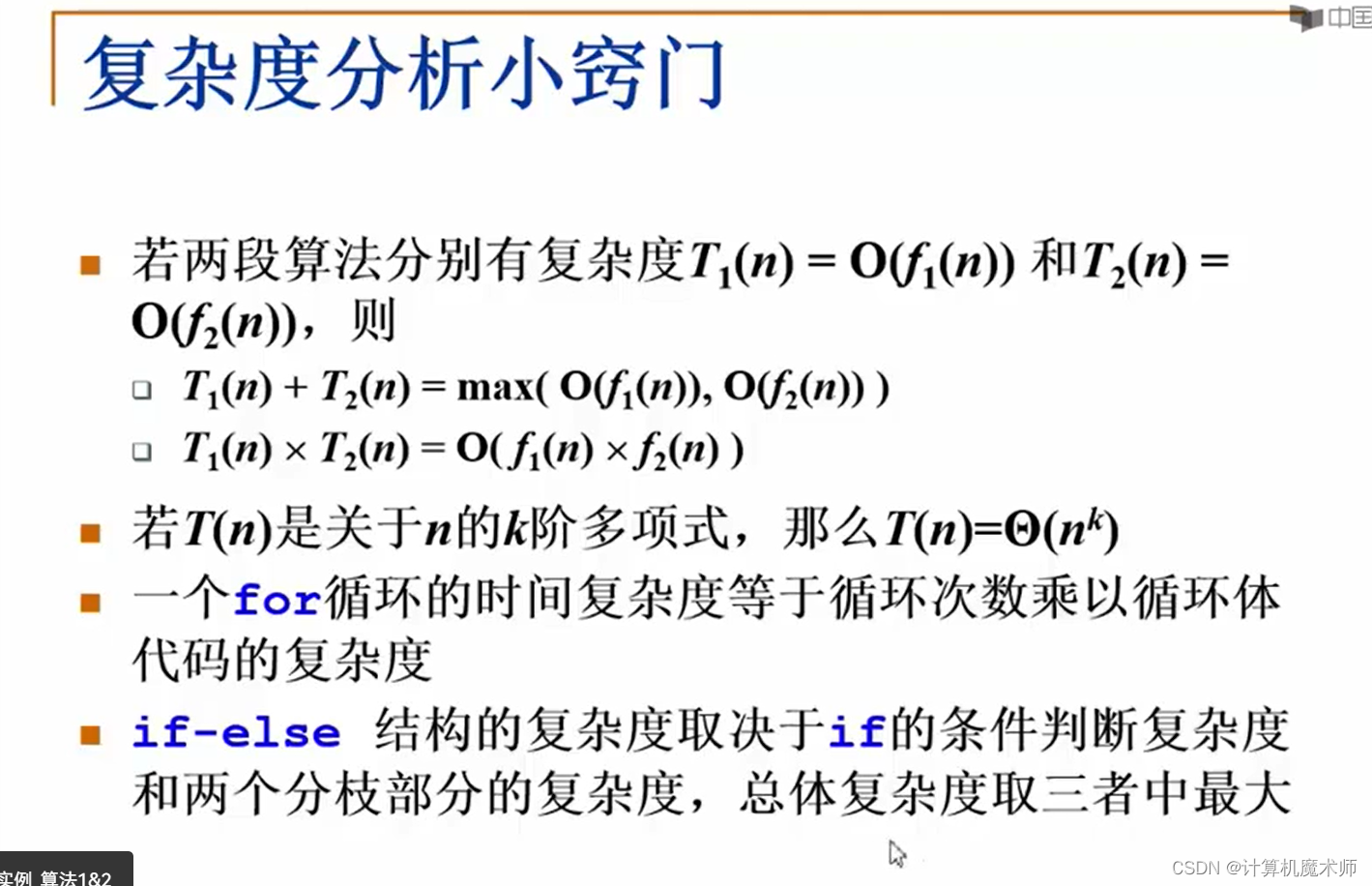## 5.3 最大子列和问题

### 算法一 - 暴力枚举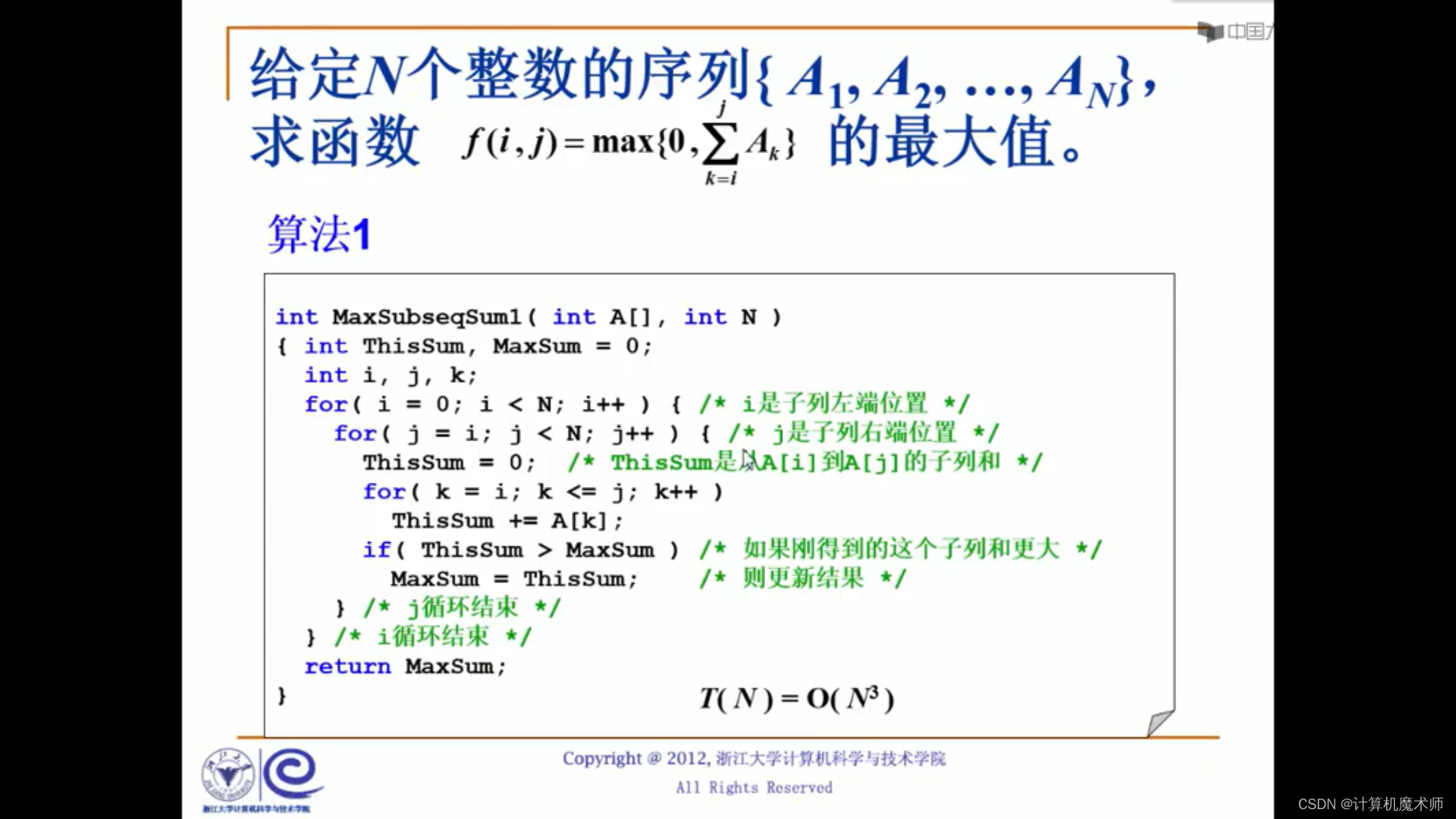### 算法二 - 减少重复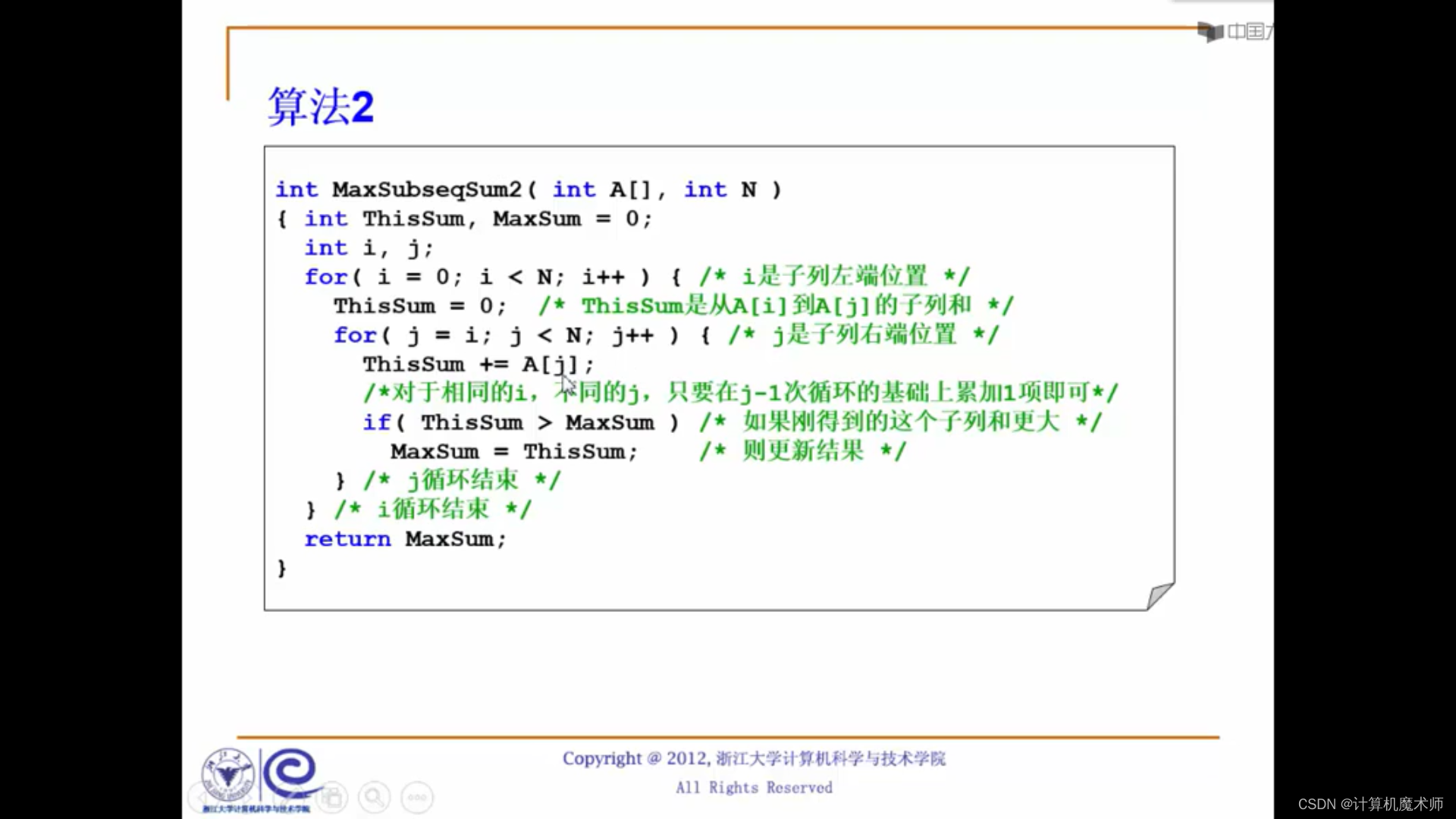### 算法三 - 分而治之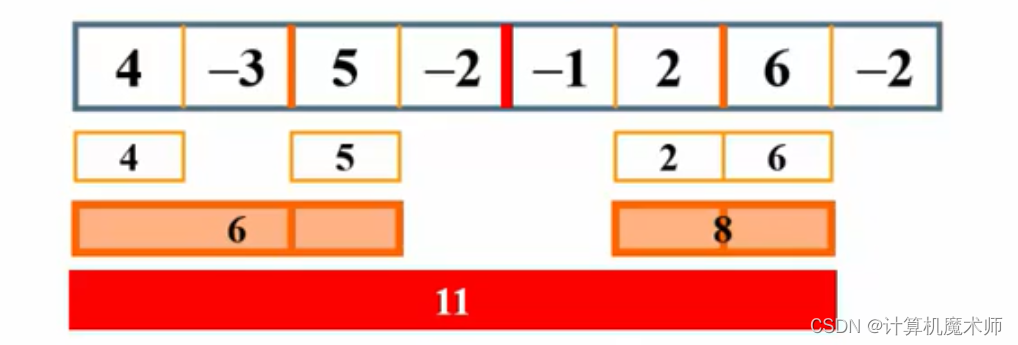``````4 5 2 6
``````

,在往上增加元素至最大值，二次选取结果为

``````6 8
``````

，在网上选取就是

``````11
``````

，可以看到方法很好理解，麻烦一点是在于程序的实现比较长。

``````     O

(

n

)

O(n)

O(n)
``````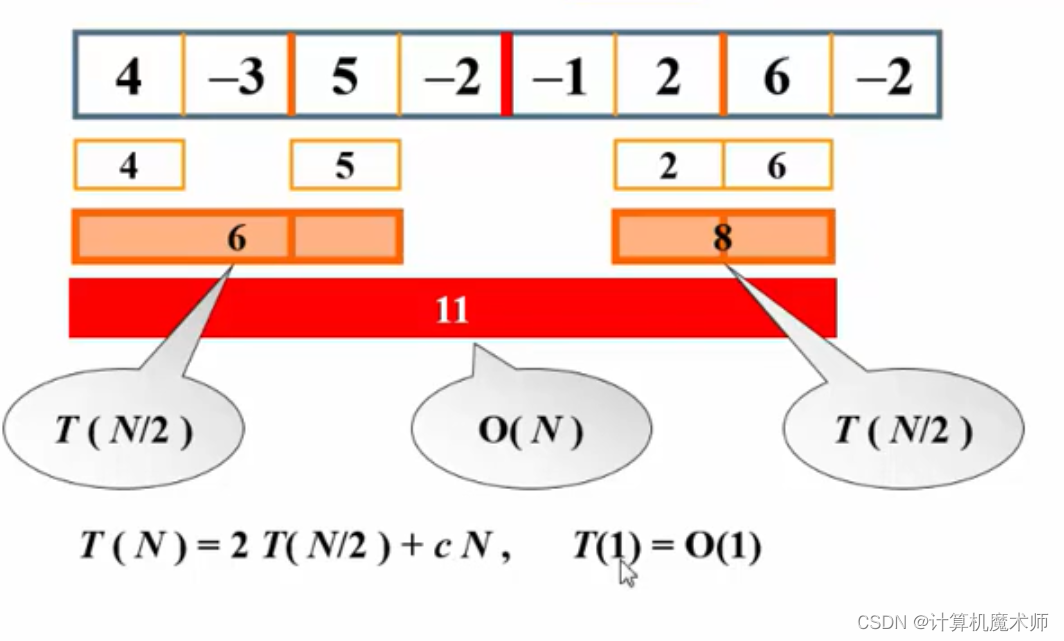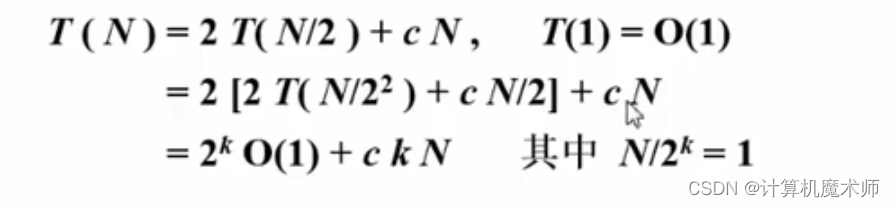``````    k

=

l

o

g

2

n

k= log_2n

k=log2​n 则

T

(

N

)

=

O

(

n

)

+

c

n

∗

l

o

g

2

n

T(N) = O(n) + cn*log_2n

T(N)=O(n)+cn∗log2​n, 在前面中提到两个复杂度式子相加，则取最大值，所以**该算法复杂度为**

n

∗

l

o

g

2

n

n*log_2n

n∗log2​n，但此时该算法还不是最快的！
``````

### 算法四 - 在线处理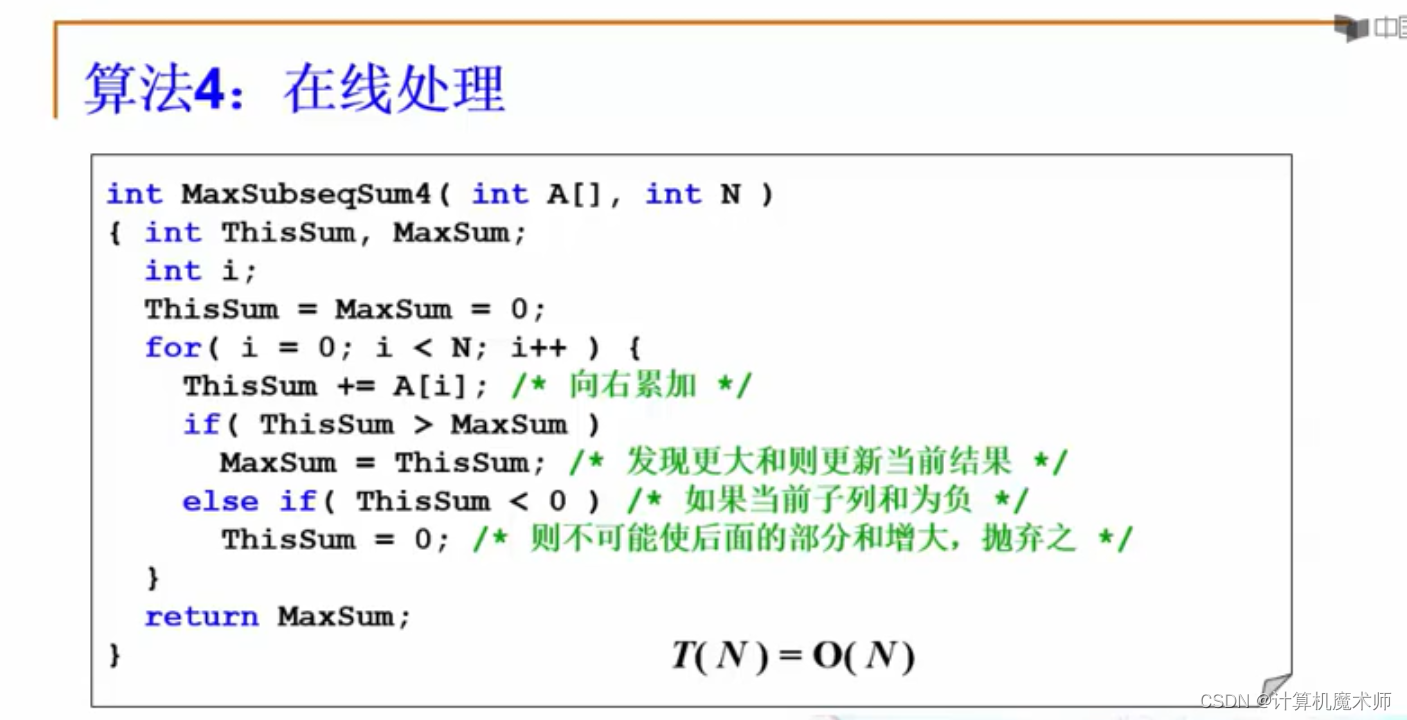``````    O

(

n

)

O(n)

O(n))
````````````  ✨谢谢你的阅读，您的点赞和收藏就是我创造的最大动力！✨
``````

### “【数据结构 | 入门】 入坑篇 （浙江大学数据结构学习笔记）”的评论:

##### 关于作者##### overfit同步小助手